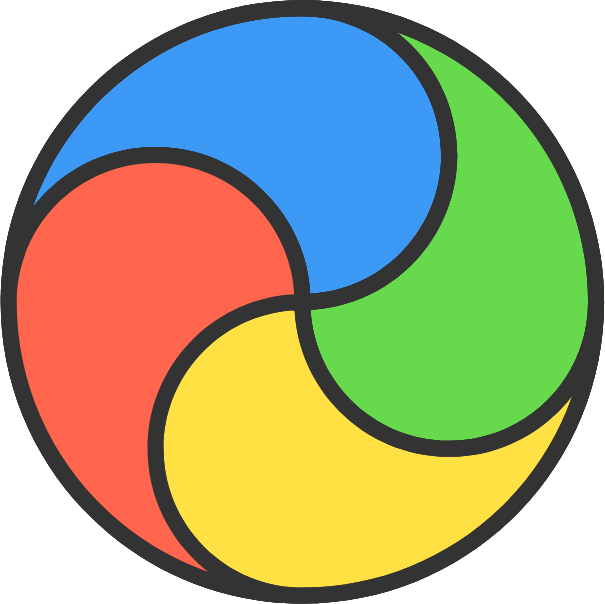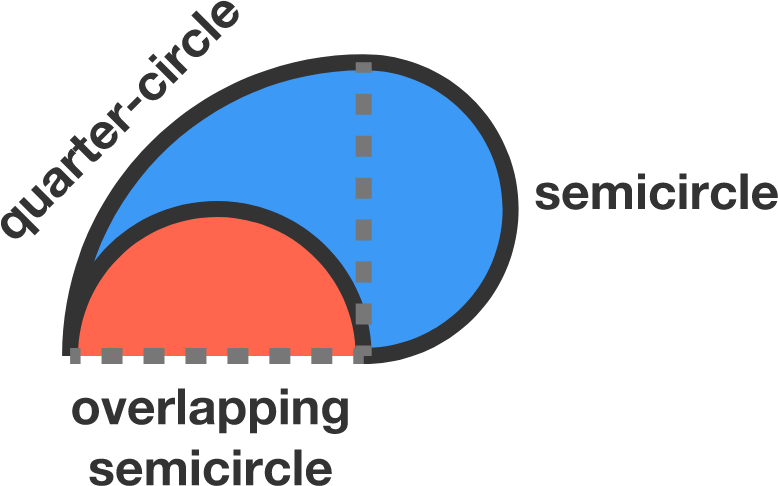# It is not a Beach Ball

Geometry Level 1

A circle is divided into four identical regions constructed out of overlapping quarter-circles and semicircles.

If the circumference of the circle is 120, then what is the perimeter of each region?The construction of each region is shown here.

×